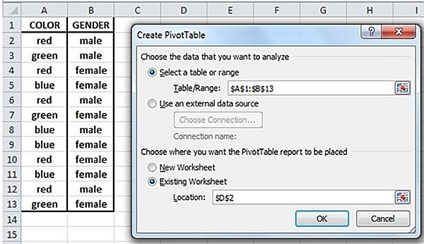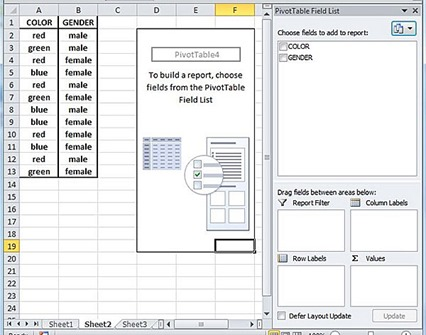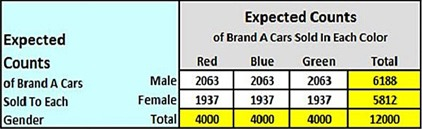# Chi-Square Independence Test in 7 Steps in Excel

The Chi-Square Independence Test is used to determine whether two categorical variables associated with the same item act independently on that item. The example presented in this section analyzes whether the gender of the purchaser of a car is independent of the color of the car. This Chi-Square Independence Test answers the question of whether gender plays a role in the color selection of a purchased car

Each item (each purchased car) has two attributes associated with it. These two attributes are the categorical variables of purchaser’s gender and color. The counts of the number of cars purchased for each unique combination of gender and color are placed in a matrix called a contingency table.

## Contingency Table

A contingency table is a two-way cross-tabulation. Each row in the contingency table is associated with one of the levels of one of the categorical attributes (such as gender) and each column is associated with one of the levels of the other categorical attribute (such as color).

The number of rows in the contingency table, r, is equal to the number of levels of the row attribute. The number of columns in the contingency table, c, is equal to the number of levels of the column attribute. The contingency table is therefore an r x c table and has r x c cells representing r x c unique combinations of levels of row and column attributes.

## Test Compares Actual vs. Expected Bin Counts

The Chi-Square Independence Test compares whether counts of the actual data for each unique combination of factors of the two variables are significantly different than the counts that would be expected if the attributes were totally independent of each other.

## Null Hypothesis

A Null Hypothesis is created which states there is no significant difference between the actual and expected counts of data for the unique combinations of levels of the two factors.

## Test Statistic

The Chi-Square Independence Test calculates a Test Statistic called a Chi-Square Statistic, Χ2. The distribution of this Test Statistic can be approximated by the Chi-Square distribution if several conditions are met.

### When to Reject Null Hypothesis

The Null Hypothesis is rejected if that Chi-Square Statistic is larger than a Critical Chi-Square Value based upon the specified alpha level and degrees of freedom associated with that test. Equivalently, the Null Hypothesis is rejected if the p value derived from the test is smaller than the specified alpha level.

## Required Assumptions

The distribution of this Test Statistic, Χ2, can be approximated by the Chi-Square distribution with degrees of freedom equal to df = (r – 1)(c – 1) if the following three conditions are met:

1) The number of cells in the contingency table (r x c) is at least 5. A 2 x 2 contingency table is not large enough. One of the two attributes must have at least 3 levels.

2) The average value of all of the expected counts is at least 5.

3) All of the expected counts equal at least 1.

## Example of Chi-Square Independence Test in Excel

We will examine whether gender and product color selection are independent of each other. A car company in the United States sold new 12,000 cars of one brand in one month. The car company recorded the gender of each customer and also the color of the car. The car was available in only three colors: red, blue, and green. The actual counts of cars purchased in that months for each unique combination of gender/color are shown as follows:Determine with 95-percent certainty the car purchaser’s gender and the selected color of the car are independent of each other.

### Step 1 – Place Actual Counts In Contingency Table

The actual counts of the number of items having each unique combination of row and column attribute level are placed into the proper cell in the r x c contingency table. In this case the counts of the number of cars associated with each unique combination of gender/color are placed into the correct cells of the 2 x 3 contingency table as follows:(Click Image To See Larger Version)

### Creating the Contingency Table From an Excel Pivot Table

The contingency table can be created with Excel’s Pivot Table tool if the data are initially presented in the following fashion as they often are:(Click Image To See Larger Version)

The Pivot Table is accessed from within the Insert tab.

Insert / Pivot Table / Pivot Table bring up the initial Pivot Table dialogue box. The table range and output location should be filled in as follows:(Click Image To See Larger Version)

Hitting OK brings up the following final Pivot Table dialogue box:(Click Image To See Larger Version)

Dragging the label Color down to the Column Labels box and to the Σ Values box and then dragging the label Gender down to the Row Labels box produces the completed Pivot Table as follows. This Pivot Table is an exact match of the contingency table containing the actual values for this data set.(Click Image To See Larger Version)

Note that the Excel Pivot Table would be an exact match for the contingency table with the actual counts that is shown again here.(Click Image To See Larger Version)

### Step 2 – Place Expected Counts In Contingency Table

The expected counts for each unique combination of levels of row/column attributes are placed into the correct cells of an identical contingency table as follows:(Click Image To See Larger Version)

The expected counts are based upon the assumption that the row and column attributed act independently of each other. The method of calculated the expected numbers based upon this assumption is shown below:(Click Image To See Larger Version)

### Step 3 – Create Null and Alternative Hypotheses

The Null Hypothesis states that there is no difference between the expected and actual counts of items for each unique combination of levels of row and column attributes. The Test Statistic, Χ2, would equal 0 in this case. The Null Hypothesis is therefore specified as follows:

H0: Χ2 = 0

The Chi-Square Statistic, Χ2, is distributed according to the Chi-Square distribution if the required assumptions for this tests that are specified in this blog article are met. The Chi-Square distribution has only one parameter: its degrees of freedom, df. The probability density function of the Chi-Square distribution calculated at x is defined as f(x,df) and can only be defined for positive values of x.

Since the Chi-Square’s PDF value f(x,df) only exists for positive values of x, the alternative hypothesis specifies that that the Chi-Square Independence Test is a one-tailed test in the right tail and is specified as follows:

H1: Χ2 > 0

### Step 4 – Verify Required Assumptions

The distribution of this Test Statistic, Χ2, can be approximated by the Chi-Square distribution with degrees of freedom equal to df = (r – 1)(c – 1) if the following three conditions are met:

1) The number of cells in the contingency table (r x c) is at least 5. The contingency table is a 2 x 3 table so this condition is met.

2) The average value of all of the expected counts is at least 5. This condition is met.

3) All of the expected counts equal at least 1. This condition is met.

### Step 5 – Calculate Chi-Square Statistic, Χ2

The Test Statistic, which is the Chi-Square Statistic, Χ2, is calculated for n = r x c unique cells in the contingency table as follows:(Click Image To See Larger Version)

This can be quickly implemented in a convenient table as follows:(Click Image To See Larger Version)

### Step 6 – Calculate Critical Chi-Square Value and p Value

The degrees of freedom for the Chi-Square Independence Test is calculated as follows:

r = number of rows = 2

c = number of columns = 3

df = (r – 1)(c – 1) = (2 – 1)(3 – 1) = 2

The Critical Chi-Square Value is calculated as follows:

Chi-Square Critical = CHISQ.INV.RT(α,df)

Chi-Square Critical = CHISQ.INV.RT(0.05,2) = 5.99

Prior to Excel 2010, the formula is calculated as follows:

Chi-Square Critical = CHIINV(α,df)

The p Value is calculated as follows:

p Value = CHISQ.DIST.RT(Chi-Square Statistic,df)

p Value = CHISQ.DIST.RT(6.17,2) = 0.0457

Prior to Excel 2010, the formula is calculated as follows:

p Value = CHIDIST(Chi-Square Statistic,df)

### Step 7 – Determine Whether To Reject Null Hypothesis

The Null Hypothesis is rejected if either of the two equivalent conditions are shown to exist:

1) Chi-Square Statistic > Critical Chi-Square Value

2) p Value < α

Both of these conditions exist as follows.

Chi-Square Statistic = 6.17

Critical Chi-Square value = 5.99

p Value = 0.0457

α = 0.05

In this case we reject the Null Hypothesis because the Chi-Square Statistic (6.17) is larger than the Critical Value (5.99) or, equivalently, the p Value (0.0457) is smaller than Alpha (0.05). A graphical representation of this problem is shown as follows in this Excel-generated graph:(Click Image To See Larger Version)

Excel Master Series Blog Directory

Statistical Topics and Articles In Each Topic

• Histograms in Excel
• Bar Chart in Excel
• Combinations & Permutations in Excel
• Normal Distribution in Excel
• t-Distribution in Excel
• Binomial Distribution in Excel
• z-Tests in Excel
• t-Tests in Excel
• Hypothesis Tests of Proportion in Excel
• Chi-Square Independence Tests in Excel
• Chi-Square Goodness-Of-Fit Tests in Excel
• F Tests in Excel
• Correlation in Excel
• Pearson Correlation in Excel
• Spearman Correlation in Excel
• Confidence Intervals in Excel
• Simple Linear Regression in Excel
• Multiple Linear Regression in Excel
• Logistic Regression in Excel
• Single-Factor ANOVA in Excel
• Two-Factor ANOVA With Replication in Excel
• Two-Factor ANOVA Without Replication in Excel
• Randomized Block Design ANOVA in Excel
• Repeated-Measures ANOVA in Excel
• ANCOVA in Excel
• Normality Testing in Excel
• Nonparametric Testing in Excel
• Post Hoc Testing in Excel
• Creating Interactive Graphs of Statistical Distributions in Excel
• Solving Problems With Other Distributions in Excel
• Optimization With Excel Solver
• Chi-Square Population Variance Test in Excel
• Analyzing Data With Pivot Tables
• SEO Functions in Excel
• Time Series Analysis in Excel
• VLOOKUP